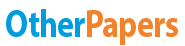# Cathode Rays and E/m Measurement Lab

Essay by   •  June 2, 2017  •  Lab Report  •  1,580 Words (7 Pages)  •  1,141 Views

## Essay Preview: Cathode Rays and E/m Measurement Lab

Report this essay
Page 1 of 7

Lab:Cathode Rays and e/m Measurement

Introduction)

Today, we are going to re-create experiment that explored the effect of electric and magnetic fields on charged particles and helped to determine the properties of the electron. In particular, we will show that the charged particles in question are negatively charged and we will calculate the ratio of the particles’ charge to the particles’ mass.

The entire experiment is built around a “cathode ray” device that produces a visible beam of electrons. The source of the electrons is a metallic filament that is heated by a current passing through it. As the filament heats up, it glows red hot and also emits electrons in a process known as “thermionic emission”, Essentially, the increased thermal motion in the filament causes electrons to “boil” off of the material. The freed electrons are then accelerated through a potential difference V to form a horizontal “beam” of electrons. The final speed of the electrons are related to the accelerating potential and their charge q as follow:

[pic 1]                          (1)

We are going to apply fields and circular motion:

The apparatus allows us to generate electric and magnetic fields that are perpendicular to the electron beam. The magnetic field is generated by the two large coils of wire, known as Helmholtz coils. This configuration of wires generates a fairly uniform magnetic field, given by:

[pic 2]                           (2)

Where I is the current passing through the coils. If this magnetic field is perpendicular to the velocity of the charged particles, then the magnitude of the force it exerts is:

[pic 3]                            (3)

This force provides the centripetal force associated with the resulting circular motion, which can be written in terms of the speed of the particles and the radius of the circular path.

[pic 4]                             (4)

The apparatus also contains “deflection plates”, which are just a pair of conductive plates across which we can apply a potential difference. This applied potential difference creates a vertical electric field which exerts an upward or downward force on the electrons.

Drive [pic 5]:

As we know that the “e” and “q” refer to the same quantity, in equation (1), we can get:

[pic 6]                                   (5)

Then, we solve the ratio of [pic 7] from equation (3) and (4), which give us:

[pic 8]                                       (6)

As we have the relationship about v from equation (5), then we plus in the v from (5) into (6):

[pic 9]                           (7)

Finally, we simplify the equation (7) to solve [pic 10]and get:

[pic 11]                                    (8)

And in this lab, the theoretical value of [pic 12] for an electron is [pic 13]

Activity 1:

To start the lab, we would setup the Helmholtz coils, e/m Apparatus and the power supply and so on as the following figure1.

[pic 14]

(Figure 1)

We turn the high voltage power supply on and then turn dial on the right until a voltage of close to but less than 300V is being supplied. It takes about 10 minutes before the electron beam becomes visible. After the electron beam becomes visible, we turn on the 3A power supply and turn the Helmholtz coil current knob on the e/m apparatus completely clockwise and then slowly turn the voltage and current limiting knobs on the power supply clockwise until a current of up to 2A is being supplied to the Helmholtz coils. Now we see a circular green beam inside the “bulb”.

...

...